# KSEEB Solutions for Class 8 Maths Chapter 6 Square and Square Roots Ex 6.3

You can Download KSEEB Solutions for Class 8 Maths Chapter 6 Square and Square Roots Ex 6.3 Questions and Answers helps you to revise the complete syllabus.

## KSEEB Solutions for Class 8 Maths Chapter 6 Square and Square Roots Ex 6.3

Question 1.
What could be the possible ‘one’s digits of the square root of each of the following numbers?
(i) 9801
(ii) 99856
(iii) 998001
(iv) 657666025
Solution:
(i) Possible one’s digit in the square root of 9801 is 1 or 9.
(ii) Possible one’s digit in the square root of 99856 is 6.
(iii) Possible one’s digit in the square root of 998001 is 1 or 9.
(iv) Possible one’s digit in the square root of 657666025 is 5.Question 2.
Without doing any calculation, find the numbers which are surely not perfect squares.
(i) 153
(ii) 257
(iii) 408
(iv) 441
Solution:
Since the number which ends with digit 2, 3, 7, 8 are surely not a perfect square.
∴ (i) 153 (ii) 257 (iii) 408 are not perfect square.

Question 3.
Find the square roots of 100 and 169 by the method of repeated subtraction.
Solution:
(I) (i) 100 – 1 = 99
(ii) 99 – 3 = 96
(iii) 96 – 5 = 91
(iv) 91 – 7 = 84
(v) 84 – 9 = 75
(vi) 75 – 11 = 64
(vii) 64 – 13 = 51
(viii) 51 – 15 = 36
(ix) 36 – 17 = 19
(x) 19 – 19 = 0
Repeated subtract of successive odd number starting from 1 gives 0 at 10th step.
Hence √100 = 10(II) (i) 169 – 1 = 168
(ii) 168 – 3 = 165
(iii) 165 – 5 = 160
(iv) 160 – 7 = 153
(v) 153 – 9 = 144
(vi) 144 – 11 = 133
(vii) 133 – 13 = 120
(viii) 120 – 15 = 105
(ix) 105 – 17 = 88
(x) 88 – 19 = 69
(xi) 69 – 21 = 48
(xii) 48 – 23 = 25
(xiii) 25 – 25 = 0
Repeated subtraction of successive odd number starting from 1 gives 0 at the 13th step
∴ Hence √169 = 13

Question 4.
Find the square roots of the following numbers by the Prime Factorisation Method.
(i) 729
(ii) 400
(iii) 1764
(iv) 4096
(v) 7744
(vi) 9604
(vii) 5929
(viii) 9216
(ix) 529
(x) 8100
Solution:
(i) √729
= $$\sqrt{3 \times 3 \times \underline{3 \times 3} \times \underline{3 \times 3}}$$
= 3 × 3 × 3
= 27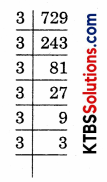(ii) √400
= $$\sqrt{\underline{2 \times 2 \times \underline{2 \times 2} \times \underline{5 \times 5}}}$$
= 2 × 2 × 5
= 20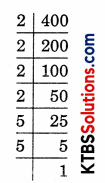(iii) √1764
= $$\sqrt{\underline{2 \times 2 \times \underline{3 \times 3} \times \underline{7 \times 7}}}$$
= 2 × 3 × 7
= 42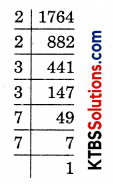(iv) √4096
= $$\sqrt{2 \times 2 \times 2 \times 2 \times 2 \times 2 \times 2 \times 2 \times 2 \times 2 \times 2 \times 2}$$
= 2 × 2 × 2 × 2 × 2 × 2
= 64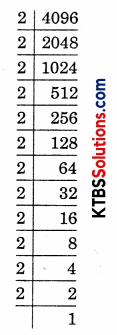(v) √7744
= $$\sqrt{2 \times 2 \times 2 \times 2 \times 2 \times 2 \times 11 \times 11}$$
= 2 × 2 × 2 × 11
= 88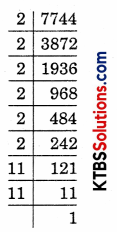(vi) √9604
= $$\sqrt{\underline{2 \times 2} \times \underline{7 \times 7} \times \underline{7 \times 7}}$$
= 2 × 7 × 7
= 98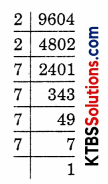(vii) √5929
= $$\sqrt{\underline{7 \times 7} \times \underline{11 \times 11}}$$
= 7 × 11
= 77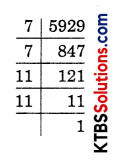(viii) √9216
= $$\sqrt{\underline{2 \times 2} \times \underline{2 \times 2} \times \underline{2 \times 2} \times \underline{2 \times 2} \times \underline{3 \times 3} \times \underline{3 \times 3}}$$
= 2 × 2 × 2 × 2 × 2 × 3
= 96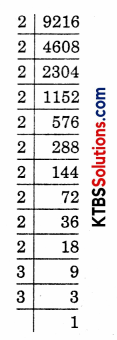(ix) √529
= $$\sqrt{23 \times 23}$$
= 23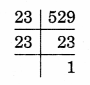(x) √8100
= $$\sqrt{\underline{2 \times 2} \times \underline{3 \times 3 \times 3 \times 3 \times 5 \times 5}}$$
= 2 × 2 × 3 × 3 × 3 × 3 × 5 × 5
= 2 × 3 × 3 × 5
= 90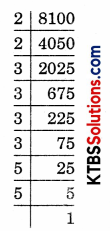Question 5.
For each of the following numbers, find the smallest whole number by which it should be multiplied, so as to get a perfect square number. Also, find the square root of the square number so obtained.
(i) 252
(ii) 180
(iii) 1008
(iv) 2028
(v) 1458
(vi) 768
Solution:
(i) 252 = 2 × 2 × 3 × 3 × 7
By prime factorisation. 7 is unpaired. Hence, to make it pair multiply by 7 then
252 × 7 = 2 × 2 × 3 × 3 × 7 × 7
√1764 = 2 × 3 × 7 = 42

(ii) 180 = 2 × 2 × 3 × 3 × 5
By prime factorisation, 5 is unpaired. Hence, multiply it by 5 pair (perfect square) then
180 × 5 = 2 × 2 × 3 × 3 × 5 × 5
√900 = 2 × 3 × 5 = 30(iii) 1008 = 2 × 2 × 2 × 2 × 3 × 3 × 7
By prime factorisations 7 is unpaired. Hence, multiply it by 7 to make its pair (perfect square) then
1008 × 7 = 2 × 2 × 2 × 2 × 3 × 3 × 7 × 7
$$\sqrt{1008 \times 7}$$ = 2 × 2 × 3 × 7 = 84

(iv) 2028 = 2 × 2 × 3 × 13 × 13 (Perfect square)
2028 × 3 = 2 × 2 × 3 × 3 × 13 × 13
√2084 = 2 × 3 × 13 = 78

(v) 1458 = 2 × 3 × 3 × 3 × 3 × 3 × 3
We see that 2 is unpaired. Hence, multiply by 2 to make is pair (perfect square)
1458 × 2 = 2 × 2 × 3 × 3 × 3 × 3 × 3 × 3
√2916 = 2 × 3 × 3 × 3 = 54

(vi) 768 = 2 × 2 × 2 × 2 × 2 × 2 × 2 × 2 × 3
We see that 3 is unpaired. Hence, multiply by 3 to make it paired (perfect square)
768 × 3 = 2 × 2 × 2 × 2 × 2 × 2 × 2 × 2 × 3 × 3
√2304 = 2 × 2 × 2 × 2 × 3 = 48Question 6.
For each of the following numbers, find the smallest whole number by which it should be divided, so as to get a perfect square. Also, find the square root of the square number so obtained
(i) 252
(ii) 2925
(iii) 396
(iv) 2645
(v) 2800
(iv) 1620
Solution:
(i) 252 = 2 × 2 × 3 × 3 × 7
7 is unpaired. Hence, divide the number 252 by 7 to make it a perfect square.
252 ÷ 7 = 36
36 = 2 × 2 × 3 × 3
√36 = 2 × 3 = 6

(ii) 2925 = 3 × 3 × 5 × 5 × 13
13 is unpaired. Hence, divide the number 2925 by 13 to make it a perfect square.
2925 ÷ 13 = 225
225 = 3 × 3 × 5 × 5
√225 = 3 × 5 = 15

(iii) 396 = 2 × 2 × 3 × 3 × 11
11 is unpaired. Hence, divide the number by 396 by 11 to make it a perfect square.
396 ÷ 11 = 36
36 = 2 × 2 × 3 × 3
√36 = 2 × 3 = 6(iv) 2645 = 5 × 23 × 23
5 is unpaired. Hence, divide the number 2645 by 5 to make it a perfect square.
2645 ÷ 5 = 529
529 = 23 × 23
√529 = 23

(v) 2800 = 2 × 2 × 2 × 2 × 5 × 5 × 7
7 is unpaired. Hence, divide the number 2800 by 7 to make it a perfect square.
2800 ÷ 7 = 400
400 = 2 × 2 × 2 × 2 × 5 × 5
√400 = 2 × 2 × 5 = 20

(vi) 1620 = 2 × 2 × 3 × 3 × 3 × 3 × 5
5 is unpaired. Hence, divide the number 1620 by 5 to make it a perfect square.
1620 ÷ 5 = 324
324 = 2 × 2 × 3 × 3 × 3 × 3
√324 = 2 × 3 × 3 = 18

Question 7.
The students of Class VIII of a school donated Rs 2401 in all, for Prime Minister’s National Relief Fund. Each student donated as many rupees as the number of students in the class. Find the number of students in the class.
Solution:
Let the number of class VIII students = x
Donation by each student = Rs x.
Total amount collected = x × x = x2
x2 = 2401
x = √2401
= $$\sqrt{7 \times 7 \times 7 \times 7}$$
= 7 × 7
= 49
∴ No. of students = 49Question 8.
2025 plants are to be planted in a garden, in such a way that each row contains as many plants as the number of rows. Find the number of rows and the number of plants in each row.
Solution:
Let the number of plants in each row = x
Number of rows = x
Total plants = x × x = x2
x2 = 2025
x = √2025
= $$\sqrt{\underline{3 \times 3} \times \underline{3 \times 3} \times \underline{5 \times 5}}$$
= 3 × 3 × 5
= 45
∴ Number of plants = 45
Number of rows 45Question 9.
Find the smallest square number that is divisible by each of the numbers 4, 9, and 10.
Solution:
Find the LCM of 4, 9, 10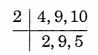LCM = 2 × 2 × 5 × 9 = 180
180 = 2 × 2 × 3 × 3 × 5
5 is unpaired. So, multiply the number 180 by 5 to make it a perfect square.
180 × 5 = 2 × 2 × 3 × 3 × 5 × 5
900 = 2 × 2 × 3 × 3 × 5 × 5
900 is a perfect square. Hence, the smallest perfect square number, divisible by 4, 9, and 10 is 900.Question 10.
Find the smallest square number that is divisible by each of the numbers 8, 15, and 20.
Solution:
Find the LCM of 8, 15, 20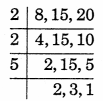LCM = 2 × 2 × 5 × 2 × 3 = 120
2, 3, 5 are unpaired. So, to make these paired, we multiply 120 by 2, 3, and 5.
120 × 2 × 3 × 5 = 2 × 2 × 5 × 5 × 2 × 2 × 3 × 3
3600 = 2 × 2 × 5 × 5 × 2 × 2 × 3 × 3
3600 is a perfect square. Hence, the smallest perfect square number, divisible by 8, 15, 20 is 3600.